Omnia vincit amor
Home -> PublicationsHome
Publications

edited volumes
Awards
Research
Teaching
Miscellaneous
Full CV [pdf]
BLOG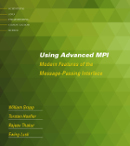Events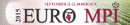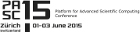Past Events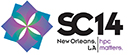Publications of Torsten Hoefler
T. Wicky, Edgar Solomonik and Torsten Hoefler:

Communication-Avoiding Parallel Algorithms for Solving Triangular Systems of Linear Equations

(In Proceedings of the 31st IEEE International Parallel & Distributed Processing Symposium (IPDPS'17), presented in Orlando, FL, USA, IEEE, May 2017)

#### Abstract

We present a new parallel algorithm for solving triangular systems with multiple right hand sides (TRSM). TRSM is used extensively in numerical linear algebra com- putations, both to solve triangular linear systems of equations as well as to compute factorizations with triangular matrices, such as Cholesky, LU, and QR. Our algorithm achieves better theoretical scalability than known alternatives, while maintaining numerical stability, via selective use of triangular matrix inversion. We leverage the fact that triangular inversion and matrix multiplication are more parallelizable than the standard TRSM algorithm. By only inverting triangular blocks along the diagonal of the initial matrix, we generalize the usual way of TRSM computation and the full matrix inversion approach. This flexibility leads to an efficient algorithm for any ratio of the number of right hand sides to the triangular matrix dimension. We provide a detailed communication cost analysis for our algorithm as well as for the recursive triangular matrix inversion. This cost analysis makes it possible to determine optimal block sizes and processor grids a priori. Relative to the best known algorithms for TRSM, our approach can require an asymptotic factor fewer messages, while performing optimal amounts of communication and computation.

#### Documents

download article: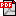#### BibTeX

 @inproceedings{comm-avoiding-triang,  author={T. Wicky and Edgar Solomonik and Torsten Hoefler},  title={{Communication-Avoiding Parallel Algorithms for Solving Triangular Systems of Linear Equations}},  year={2017},  month={May},  booktitle={Proceedings of the 31st IEEE International Parallel \& Distributed Processing Symposium (IPDPS'17)},  location={Orlando, FL, USA},  publisher={IEEE},  source={http://www.unixer.de/~htor/publications/},}